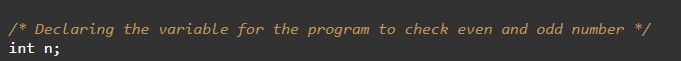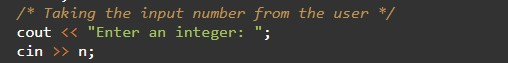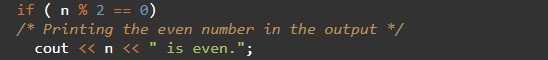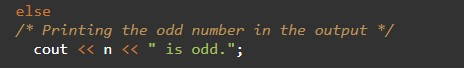# C++ Programs to Check Even and Odd Number

In this tutorial you will learn about the C++ Programs to Check Even and Odd Number and its application with practical example.

## C++ Program to Check Even and Odd Number

In this tutorial, we will learn to create a C++ program that will Check Even and Odd numbers using C++ programming.

## Prerequisites

Before starting with this tutorial we assume that you are best aware of the following C++ programming topics:

• Operators in C++ Programming.
• Basic Input and Output function in C++ Programming.
• Basic C++ programming.
• Conditional Statements in C++ programming.
• Arithmetic operations in C++ Programming.

## What is an even and odd number?

Even numbers are those numbers that are completely divisible by 2. which means the reminder should be zero. And odd numbers are those numbers that cannot be completely divisible by 2.

## Program to Check Even and Odd Number

In this program, we will take an integer input number from the user, and then we will use it in the program with conditional statements to find whether the number is even or odd. As per the program calculations.

With the help of this program, we can Check Even and Odd numbers.

## Output:-In the above program, we have first initialized the required variable.• n = it will hold the integer value.

Input number from the user.Checking if even number.Checking for an odd number.In this tutorial we have learn about the C++ Programs to Check Even and Odd Number and its application with practical example. I hope you will like this tutorial.